Courses

# Memory Management And Virtual Memory MCQ Quiz - 1

## 30 Questions MCQ Test RRB JE for Computer Science Engineering | Memory Management And Virtual Memory MCQ Quiz - 1

Description
This mock test of Memory Management And Virtual Memory MCQ Quiz - 1 for Computer Science Engineering (CSE) helps you for every Computer Science Engineering (CSE) entrance exam. This contains 30 Multiple Choice Questions for Computer Science Engineering (CSE) Memory Management And Virtual Memory MCQ Quiz - 1 (mcq) to study with solutions a complete question bank. The solved questions answers in this Memory Management And Virtual Memory MCQ Quiz - 1 quiz give you a good mix of easy questions and tough questions. Computer Science Engineering (CSE) students definitely take this Memory Management And Virtual Memory MCQ Quiz - 1 exercise for a better result in the exam. You can find other Memory Management And Virtual Memory MCQ Quiz - 1 extra questions, long questions & short questions for Computer Science Engineering (CSE) on EduRev as well by searching above.
QUESTION: 1

### Which of the following is NOT an advantage of using shared, dynamically linked libraries as opposed to using statically linked libraries ?

Solution:

Refer Static and Dynamic Libraries In Non-Shared (static) libraries, since library code is connected at compile time, the final executable has no dependencies on the the library at run time i.e. no additional run-time loading costs, it means that you don’t need to carry along a copy of the library that is being used and you have everything under your control and there is no dependency.

QUESTION: 2

### A processor uses 2-level page tables for virtual to physical address translation. Page tables for both levels are stored in the main memory. Virtual and physical addresses are both 32 bits wide. The memory is byte addressable. For virtual to physical address translation, the 10 most significant bits of the virtual address are used as index into the first level page table while the next 10 bits are used as index into the second level page table. The 12 least significant bits of the virtual address are used as offset within the page. Assume that the page table entries in both levels of page tables are 4 bytes wide. Further, the processor has a translation look-aside buffer (TLB), with a hit rate of 96%. The TLB caches recently used virtual page numbers and the corresponding physical page numbers. The processor also has a physically addressed cache with a hit rate of 90%. Main memory access time is 10 ns, cache access time is 1 ns, and TLB access time is also 1 ns. Assuming that no page faults occur, the average time taken to access a virtual address is approximately (to the nearest 0.5 ns)

Solution:

The possibilities are
TLB Hit*Cache Hit +
TLB Hit*Cache Miss +
TLB Miss*Cache Hit +
TLB Miss*Cache Miss
= 0.96*0.9*2 +
0.96*0.1*12 + 0.04*0.9*22 +
0,04*0.1*32
= 3.8
≈ 4

Why 22 and 32? 22 is because when TLB miss occurs it takes 1ns and the for the physical address it has to go through two level page tables which are in main memory and takes 2 memory access and the that page is found in cache taking 1 ns which gives a total of 22

QUESTION: 3

Solution:

Breakup of given addresses into bit form:- 32bits are broken up as 10bits (L2) | 10bits (L1) | 12bits (offset)
first code page:
0x00000000 = 0000 0000 00 | 00 0000 0000 | 0000 0000 0000
so next code page will start from 0x00001000 = 0000 0000 00 | 00 0000 0001 | 0000 0000 0000
first data page:
0x00400000 = 0000 0000 01 | 00 0000 0000 | 0000 0000 0000
so next data page will start from 0x00401000 = 0000 0000 01 | 00 0000 0001 | 0000 0000 0000
only one stack page:
0xFFFFF000 = 1111 1111 11 | 11 1111 1111 | 0000 0000 0000
Now, for second level page table, we will just require 1 Page which will contain following 3 distinct entries i.e. 0000 0000 00, 0000 0000 01, 1111 1111 11. Now, for each of these distinct entries, we will have 1-1 page in Level-1.
Hence, we will have in total 4 pages and page size = 2^12 = 4KB.
Therefore, Memory required to store page table = 4*4KB = 16KB.

QUESTION: 4

Which of the following is not a form of memory?

Solution:

Instruction Cache - Used for storing instructions that are frequently used Instruction Register - Part of CPU's control unit that stores the instruction currently being executed Instruction Opcode - It is the portion of a machine language instruction that specifies the operation to be performed Translation Lookaside Buffer - It is a memory cache that stores recent translations of virtual memory to physical addresses for faster access. So, all the above except Instruction Opcode are memories. Thus, C is the correct choice. Please comment below if you find anything wrong in the above post.

QUESTION: 5

The optimal page replacement algorithm will select the page that

Solution:

The optimal page replacement algorithm will select the page whose next occurrence will be after the longest time in future. For example, if we need to swap a page and there are two options from which we can swap, say one would be used after 10s and the other after 5s, then the algorithm will swap out the page that would be required 10s later. Thus, B is the correct choice. Please comment below if you find anything wrong in the above post.

QUESTION: 6

Dynamic linking can cause security concerns because:

Solution:

Static Linking and Static Libraries is the result of the linker making copy of all used library functions to the executable file. Static Linking creates larger binary files, and need more space on disk and main memory. Examples of static libraries (libraries which are statically linked) are, .a files in Linux and .lib files in Windows. Dynamic linking and Dynamic Libraries Dynamic Linking doesn’t require the code to be copied, it is done by just placing name of the library in the binary file. The actual linking happens when the program is run, when both the binary file and the library are in memory. Examples of Dynamic libraries (libraries which are linked at run-time) are, .so in Linux and .dll in Windows. In Dynamic Linking,the path for searching dynamic libraries is not known till runtime

QUESTION: 7

Which of the following statements is false?

Solution:
QUESTION: 8

The process of assigning load addresses to the various parts of the program and adjusting the code and date in the program to reflect the assigned addresses is called

Solution:

Relocation of code is the process done by the linker-loader when a program is copied from external storage into main memory.
A linker relocates the code by searching files and libraries to replace symbolic references of libraries with actual usable addresses in memory before running a program.

Thus, option (C) is the answer.

Please comment below if you find anything wrong in the above post.

QUESTION: 9

Where does the swap space reside?

Solution:

Swap space is an area on disk that temporarily holds a process memory image. When memory is full and process needs memory, inactive  parts of process are put in swap space of disk.

QUESTION: 10

Consider a virtual memory system with FIFO page replacement policy. For an arbitrary page access pattern, increasing the number of page frames in main memory will

Solution:
QUESTION: 11

Consider a machine with 64 MB physical memory and a 32-bit virtual address space. If the page size is 4KB, what is the approximate size of the page table?

Solution:
QUESTION: 12

Suppose the time to service a page fault is on the average 10 milliseconds, while a memory access takes 1 microsecond. Then a 99.99% hit ratio results in average memory access time of

Solution:

If any page request comes it will first search into page table, if present, then it will directly fetch the page from memory, thus in this case time requires will be only memory access time. But if required page will not be found, first we have to bring it out and then go for memory access. This extra time is called page fault service time. Let hit ratio be p , memory access time be t1 , and page fault service time be t2.

Hence, average memory access time = p*t1 + (1-p)*t2
=(99.99*1 + 0.01*(10*1000 + 1))/100 =1.9999 *10^-6 sec

QUESTION: 13

Consider a system with byte-addressable memory, 32 bit logical addresses, 4 kilobyte page size and page table entries of 4 bytes each. The size of the page table in the system in megabytes is ___________

Solution:

Number of entries in page table = 232 / 4Kbyte
= 232 / 212
= 220

Size of page table = (No. page table entries)*(Size of an entry)
= 220 * 4 bytes
= 222 = 4 Megabytes

QUESTION: 14

A computer system implements a 40 bit virtual address, page size of 8 kilobytes, and a 128-entry translation look-aside buffer (TLB) organized into 32 sets each having four ways. Assume that the TLB tag does not store any process id. The minimum length of the TLB tag in bits is _________

Solution:

Total virtual address size = 40
Since there are 32 sets, set offset = 5
Since page size is 8kilobytes, word offset = 13
Minimum tag size = 40 - 5- 13 = 22

QUESTION: 15

Consider six memory partitions of size 200 KB, 400 KB, 600 KB, 500 KB, 300 KB, and 250 KB, where KB refers to kilobyte. These partitions need to be allotted to four processes of sizes 357 KB, 210 KB, 468 KB and 491 KB in that order. If the best fit algorithm is used, which partitions are NOT allotted to any process?

Solution:

Best fit allocates the smallest block among those that are large enough for the new process. So the memory blocks are allocated in below order.
357 ---> 400
210 ---> 250
468 ---> 500
491 ---> 600

Sot the remaining blocks are of 200 KB and 300 KB

QUESTION: 16

A Computer system implements 8 kilobyte pages and a 32-bit physical address space. Each page table entry contains a valid bit, a dirty bit three permission bits, and the translation. If the maximum size of the page table of a process is 24 megabytes, the length of the virtual address supported by the system is _______________ bits

Solution:

Max size of virtual address can be calculated by calculating maximum number of page table entries.
Maximum Number of page table entries can be calculated using given maximum page table size and size of a page table entry.
Given maximum page table size = 24 MB
Let us calculate size of a page table entry.
A page table entry has following number of bits.
1 (valid bit) +
1 (dirty bit) +
3 (permission bits) +
x bits to store physical address space of a page.

Value of x = (Total bits in physical address) - (Total bits for addressing within a page)
Since size of a page is 8 kilobytes, total bits needed within a page is 13.
So value of x = 32 - 13 = 19

Putting value of x, we get size of a page table entry = 1 + 1 + 3 + 19 = 24bits.
Number of page table entries
= (Page Table Size) / (An entry size)
= (24 megabytes / 24 bits)
= 223

= (Number of page table entries) * (Page Size)
= 223 * 8 kilobits
= 236
Therefore, length of virtual address space = 36

QUESTION: 17

Which one of the following is NOT shared by the threads of the same process?

Solution:

Threads can not share stack (used for maintaining function calls) as they may have their individual function call sequence.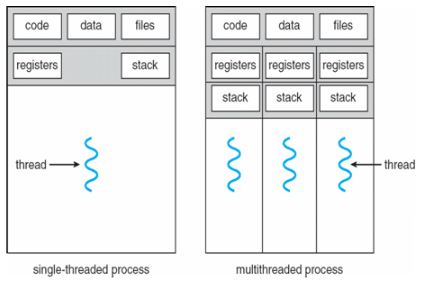QUESTION: 18

Consider a fully associative cache with 8 cache blocks (numbered 0-7) and the following sequence of memory block requests: 4, 3, 25, 8, 19, 6, 25, 8, 16, 35, 45, 22, 8, 3, 16, 25, 7 If LRU replacement policy is used, which cache block will have memory block 7?

Solution:

Block size is =8 Given 4, 3, 25, 8, 19, 6, 25, 8, 16, 35, 45, 22, 8, 3, 16, 25, 7 So from 0 to 7 ,we have

• 4 3 25 8 19 6 16 35    //25,8 LRU so next 16,35 come in the block.
•  45 3 25 8 19 6 16 35
• 45 22 25 8 19 6 16 35
• 45 22 25 8 19 6 16 35
• 45 22 25 8 3 6 16 35     //16 and 25 already there
• 45 22 25 8 3 7 16 35   //7 in 5th block Therefore , answer is  B
QUESTION: 19

The storage area of a disk has innermost diameter of 10 cm and outermost diameter of 20 cm. The maximum storage density of the disk is 1400bits/cm. The disk rotates at a speed of 4200 RPM. The main memory of a computer has 64-bit word length and 1µs cycle time. If cycle stealing is used for data transfer from the disk, the percentage of memory cycles stolen for transferring one word is

Solution:

Inner most diameter = 10 cm Storage density = 1400 bits/cm
Capacity of each track : = 3.14 * diameter * density = 3.14 * 10 * 1400 = 43960 bits
Rotational latency = 60/4200 =1/70 seconds
It is given that the main memory of a computer has 64-bit word length and 1µs cycle time.
Data transferred in 1 sec = 64 * 106 bits Data read by disk in 1 sec = 43960 * 70 = 3.08 * 106 bits
Total memory cycle = (3.08 * 106) / (64 * 106) = 5%

Thus, option (C) is correct.

Please comment below if you find anything wrong in the above post

QUESTION: 20

A disk has 200 tracks (numbered 0 through 199). At a given time, it was servicing the request of reading data from track 120, and at the previous request, service was for track 90. The pending requests (in order of their arrival) are for track numbers. 30 70 115 130 110 80 20 25. How many times will the head change its direction for the disk scheduling policies SSTF(Shortest Seek Time First) and FCFS (First Come Fist Serve)

Solution:

According to Shortest Seek Time First: 90-> 120-> 115-> 110-> 130-> 80-> 70-> 30-> 25-> 20 Change of direction(Total 3); 120->15; 110->130; 130->80 According to First Come First Serve: 90-> 120-> 30-> 70-> 115-> 130-> 110-> 80-> 20-> 25 Change of direction(Total 4); 120->30; 30->70; 130->110;20->25 Therefore,Answer is C

QUESTION: 21

In a virtual memory system, size of virtual address is 32-bit, size of physical address is 30-bit, page size is 4 Kbyte and size of each page table entry is 32-bit. The main memory is byte addressable. Which one of the following is the maximum number of bits that can be used for storing protection and other information in each page table entry?

Solution:

Virtual memory = 232 bytes Physical memory = 230 bytes
Page size = Frame size = 4 * 103 bytes = 22 * 210 bytes = 212 bytes
Number of frames = Physical memory / Frame size = 230/212 = 218
Therefore, Numbers of bits for frame = 18 bits
Page Table Entry Size = Number of bits for frame + Other information Other information = 32 - 18 = 14 bits

Thus, option (D) is correct.

Please comment below if you find anything wrong in the above post.

QUESTION: 22

In a particular Unix OS, each data block is of size 1024 bytes, each node has 10 direct data block addresses and three additional addresses: one for single indirect block, one for double indirect block and one for triple indirect block. Also, each block can contain addresses for 128 blocks. Which one of the following is approximately the maximum size of a file in the file system?

Solution: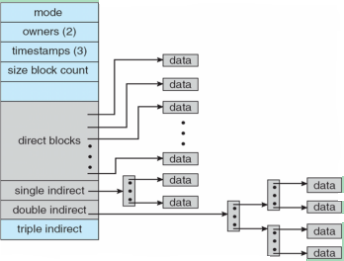The diagram is taken from Operating System Concept book.
Maximum size of the File System = Summation of size of all the data blocks whose addresses belongs to the file.
Given: Size of 1 data block = 1024 Bytes No. of addresses which 1 data block can contain = 128

Now, Maximum File Size can be calculated as:
10 direct addresses of data blocks = 10*1024
1 single indirect data block = 128*1024
1 doubly indirect data block = 128*128*1024
1 triple indirect data block = 128*128*128*1024

Hence,
Max File Size = 10*1024 + 128*1024 + 128*128*1024 + 128*128*128*1024 Bytes
= 2113674*1024 Bytes
= 2.0157 GB ~ 2GB

QUESTION: 23

A two-way switch has three terminals a, b and c. In ON position (logic value 1), a is connected to b, and in OFF position, a is connected to c. Two of these two-way switches S1 and S2 are connected to a bulb as shown below.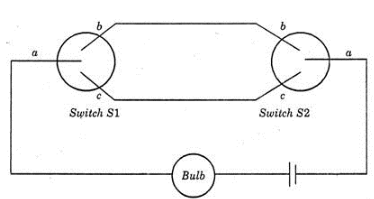Which of the following expressions, if true, will always result in the lighting of the bulb ?

Solution:

If we draw truth table of the above circuit,it'll be
S1              S2
Bulb
0         0
On 0            1
Off 1            0
Off 1            1
On = (S1⊕ S2)'

QUESTION: 24

Consider a 2-way set associative cache memory with 4 sets and total 8 cache blocks (0-7) and a main memory with 128 blocks (0-127). What memory blocks will be present in the cache after the following sequence of memory block references if LRU policy is used for cache block replacement. Assuming that initially the cache did not have any memory block from the current job? 0 5 3 9 7 0 16 55

Solution:

2-way set associative cache memory, .i.e K = 2.
No of sets is given as 4, i.e. S = 4 (numbered 0 - 3)
No of blocks in cache memory is given as 8, i.e. N =8 (numbered from 0 -7)
Each set in cache memory contains 2 blocks.
The number of blocks in the main memory is 128, i.e  M = 128. (numbered from 0 -127)

A referred block numbered X of the main memory is placed in the set numbered (X mod S) of the the cache memory. In that set, the block can be placed at any location, but if the set has already become full, then the current referred block of the main memory should replace a block in that set according to some replacement policy. Here the replacement policy is LRU ( i.e. Least Recently Used block should be replaced with currently referred block).

X (Referred block no) and the corresponding Set values are as follows:

X-->set no (X mod 4)

0--->0   (block 0 is placed in set 0, set 0 has 2 empty block locations, block 0 is placed in any one of them)
5--->1   (block 5 is placed in set 1, set 1 has 2 empty block locations, block 5 is placed in any one of them)
3--->3  (block 3 is placed in set 3, set 3 has 2 empty block locations, block 3 is placed in any one of them)
9--->1  (block 9 is placed in set 1, set 1 has currently 1 empty block location, block 9 is placed in that, now set 1 is full, and block 5 is the least recently used block)
7--->3  (block 7 is placed in set 3, set 3 has 1 empty block location, block 7 is placed in that, set 3 is full now, and block 3 is the least recently used block)
0---> block 0 is referred again, and it is present in the cache memory in set 0, so no need to put again this block into the cache memory.
16--->0  (block 16 is placed in set 0, set 0 has 1 empty block location, block 0 is placed in that, set 0 is full now, and block 0 is the LRU one)
55--->3 (block 55 should be placed in set 3, but set 3 is full with block 3 and 7, hence need to replace one block with block 55, as block 3 is the least recently used block in the set 3, it is replaced with block 55.

Hence the main memory blocks present in the cache memory are : 0, 5, 7, 9, 16, 55 .(Note: block 3 is not present in the cache memory, it was replaced with block 55) Read the following articles to learn more related to the above question: Cache Memory Cache Organization | Introduction.

QUESTION: 25

A disk has 8 equidistant tracks. The diameters of the innermost and outermost tracks are 1 cm and 8 cm respectively. The innermost track has a storage capacity of 10 MB. What is the total amount of data that can be stored on the disk if it is used with a drive that rotates it with (i) Constant Linear Velocity (ii) Constant Angular Velocity?

Solution:

Constant linear velocity :
Diameter of inner track = d = 1cm Circumference of inner track : = 2 * 3.14 * (d/2) = 3.14 cm
Storage capacity = 10 MB (given) Circumference of all equidistant tracks : = 2 * 3.14 *(0.5 + 1 + 1.5 + 2 + 2.5 + 3+ 3.5 + 4) = 113.14cm
Here, 3.14 cm holds 10 MB. Therefore, 1 cm holds 3.18 MB. 113.14 cm holds 113.14 * 3.18 = 360 MB. Total amount of data that can be stored on the disk = 360 MB

Constant angular velocity :
In case of CAV, the disk rotates at a constant angular speed. Same rotation time is taken by all the tracks. Total amount of data that can be stored on the disk = 8 * 10 = 80 MB

Thus, option (D) is correct.

Please comment below if you find anything wrong in the above post.

QUESTION: 26

Consider a computer system with 40-bit virtual addressing and page size of sixteen kilobytes. If the computer system has a one-level page table per process and each page table entry requires 48 bits, then the size of the per-process page table is _________megabytes.

Solution:

Size of memory = 240 Page size = 16KB = 214   No of pages= size of Memory/ page size = 240 / 214 = 226 Size of page table = 226 * 48/8 bytes = 26*6 MB =384 MB   Thus, A is the correct choice.

QUESTION: 27

Consider a computer system with ten physical page frames. The system is provided with an access sequence a1, a2, ..., a20, a1, a2, ..., a20), where each ai number. The difference in the number of page faults between the last-in-first-out page replacement policy and the optimal page replacement policy is __________
[Note that this question was originally Fill-in-the-Blanks question]

Solution:

LIFO stands for last in, first out a1 to a10 will result in page faults, So 10 page faults from a1 to a10. Then a11 will replace a10(last in is a10), a12 will replace a11 and so on till a20, so 10 page faults from a11 to a20 and a20 will be top of stack and a9…a1 are remained as such. Then a1 to a9 are already there. So 0 page faults from a1 to a9. a10 will replace a20, a11 will replace a10 and so on. So 11 page faults from a10 to a20. So total faults will be 10+10+11 = 31.
Optimal a1 to a10 will result in page faults, So 10 page faults from a1 to a10. Then a11 will replace a10 because among a1 to a10, a10 will be used later, a12 will replace a11 and so on. So 10 page faults from a11 to a20 and a20 will be top of stack and a9…a1 are remained as such. Then a1 to a9 are already there. So 0 page faults from a1 to a9. a10 will replace a1 because it will not be used afterwards and so on, a10 to a19 will have 10 page faults. a20 is already there, so no page fault for a20. Total faults 10+10+10 = 30. Difference = 1

QUESTION: 28

In which one of the following page replacement algorithms it is possible for the page fault rate to increase even when the number of allocated frames increases?

Solution:

In some situations FIFO page replacement gives more page faults when increasing the number of page frames. This situation is Belady’s anomaly. Belady’s anomaly proves that it is possible to have more page faults when increasing the number of page frames while using the First in First Out (FIFO) page replacement algorithm. For example, if we consider reference string 3 2 1 0 3 2 4 3 2 1 0 4 and 3 slots, we get 9 total page faults, but if we increase slots to 4, we get 10 page faults.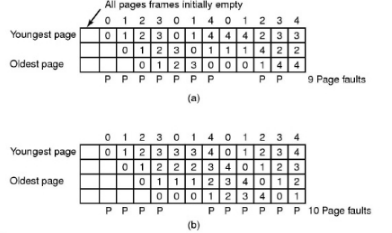QUESTION: 29

The address sequence generated by tracing a particular program executing in a pure demand paging system with 100 bytes per page is
0100, 0200, 0430, 0499, 0510, 0530, 0560, 0120, 0220, 0240, 0260, 0320, 0410.
Suppose that the memory can store only one page and if x is the address which causes a page fault then the bytes from addresses x to x + 99 are loaded on to the memory.

Q. How many page faults will occur ?

Solution: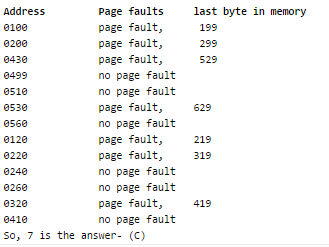QUESTION: 30

A paging scheme uses a Translation Look-aside Buffer (TLB). A TLB-access takes 10 ns and a main memory access takes 50 ns. What is the effective access time(in ns) if the TLB hit ratio is 90% and there is no page-fault?

Solution:

Effective access time = hit ratio * time during hit + miss ratio * time during miss TLB time = 10ns, Memory time = 50ns Hit Ratio= 90% E.A.T. = (0.90)*(60)+0.10*110 =65